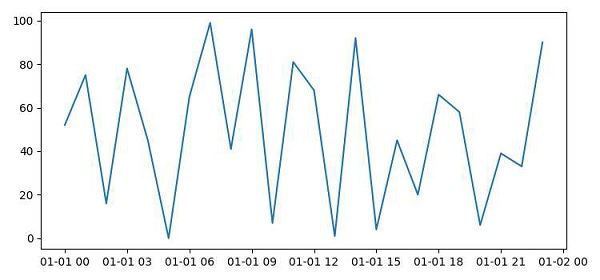# How to plot a time series in Python?

To plot a time series in Python using matplotlib, we can take the following steps −

• Create x and y points, using numpy.

• Plot the created x and y points using the plot() method.

• To display the figure, use the show() method.

## Example

import matplotlib.pyplot as plt
import datetime
import numpy as np
plt.rcParams["figure.figsize"] = [7.50, 3.50]
plt.rcParams["figure.autolayout"] = True
x = np.array([datetime.datetime(2021, 1, 1, i, 0) for i in range(24)])
y = np.random.randint(100, size=x.shape)
plt.plot(x, y)
plt.show()

## Output